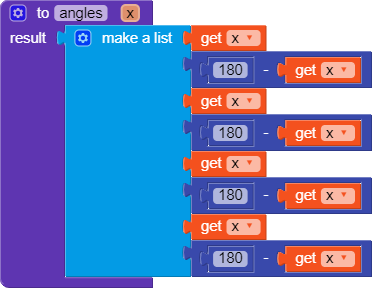# Daily Challenge #65

Daily Challenge
You are provided with an angle X(Take any random value).
You have to give out all other angles of intersection in list form
For Example(X=70)
The result should be like (70,110,70,110,70,110,70,110)

Rules

Try to do it with only one linear pair.

It might be a bit easy. But It was my first try

1 Like

What kind of?

Which angles should be in which order?

Also, very good math DC!

1 Like

It would be nice to provide an example. Anyways, here is my solution in Python, I will post Kodular blocks in a few minutes.

My Solution``````x = int(input())
y = 180-x
list = [x,y,x,y,x,y,x,y]
print(list)
``````

EDIT: Kodular blocks posted.

Toooooo fast!

1 Like

Try with only one linear pair and you have to give 8 angles not four.

1 Like

Oh, right. Hang on.

Ok, so just repeat the four angles? lmao

2 Likes

Yes just repeat the four angles.

1 Like

Here’s my

Solution

It can be that it has a mistake, because I have no companion at the moment so I couldn’t test it.Aaand also I don’t know about emulators.1 Like

Let me clear it out you need to use properties if intersection.
When a line intersects two parallel lines, take reference from here:
https://www.mathplanet.com/education/pre-algebra/introducing-geometry/angles-and-parallel-lines

Isn’t this juuust what we’ve done?

No, you can’t use linear pair* more than once.
*Sum of angles = 180

Yes, this is a better and faster and easier solution, @Mateja. Of course I didn’t get this idea.wdym? I don’t know much mathematic words in english, sorry.

1 Like

*Sum of angles = 180 degrees

But as I said he is subtracting it from 180 every time.
But according to the rules you can use that only once.

Oooh. I understand now. Thx!

1 Like

What’s with my solution? I just never used

, So what?

That’s pretty much the same thing
Just see this
x=180-y or
2x=360-2y. These are just the same equations.
You need to use mathematical properties to find out the solution.

Yes but I am using variable, so I am not repeating everything2 Likes

Yes, both the solutions are right. So I am marking the first one as solution.

1 Like